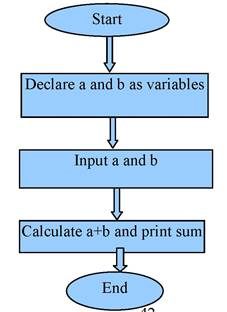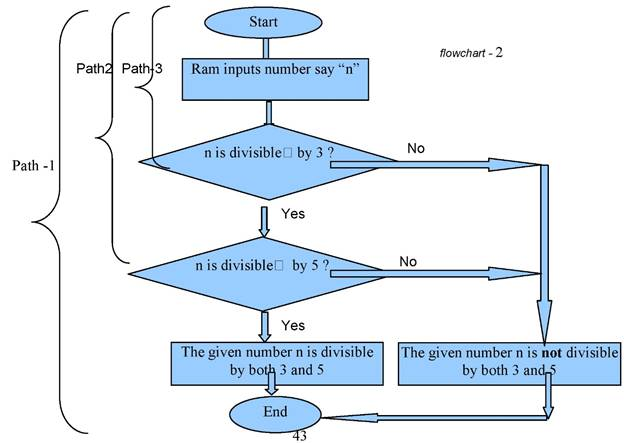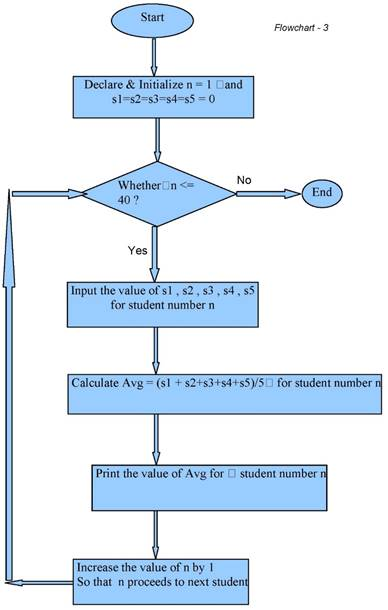1. /
2. CBSE
3. /
4. Class 11
5. /
6. Programming in C P...

# Programming in C P P class 11 Notes Computer Science### myCBSEguide App

Download the app to get CBSE Sample Papers 2023-24, NCERT Solutions (Revised), Most Important Questions, Previous Year Question Bank, Mock Tests, and Detailed Notes.

CBSE Programming in C P P class 11 Notes Computer Science in PDF are available for free download in myCBSEguide mobile app. The best app for CBSE students now provides Programming in C P P class 11 Notes Computer Science latest chapter wise notes for quick preparation of CBSE exams and school based annual examinations. Class 11 Computer Science notes on Chapter 4 Programming in C P P class 11 Notes Computer Science are also available for download in CBSE Guide website.

## CBSE Guide Programming in C P P class 11 Notes Computer Science

CBSE guide notes are the comprehensive notes which covers the latest syllabus of CBSE and NCERT. It includes all the topics given in NCERT class 11 Computer Science text book. Users can download CBSE guide quick revision notes from myCBSEguide mobile app and my CBSE guide website.

## 11 Computer Science notes Chapter 4 Programming in C P P

Download CBSE class 11th revision notes for chapter 4 Programming in C P P in PDF format for free. Download revision notes for Programming in C P P class 11 Notes and score high in exams. These are the Programming in C P P class 11 Notes Computer Science prepared by team of expert teachers. The revision notes help you revise the whole chapter 4 in minutes. Revision notes in exam days is one of the best tips recommended by teachers during exam days.

CBSE Class 11 Computer Science
Revision Notes
Programming in C P P class 11 Notes Computer Science
Chapter -4

Investigation of Programming Construct in C++.

OBJECTIVES:

• to emphasize the programming construct available in C++

• to investigate each and every programming construct emphasizing the situation where they can be used perfectly.

• to demonstrate few working program in C++ using the constructs.

1.1 Why and What of Constructs in C++ programming

1.1.1: Flow of logic

You have seen in the previous unit that a program is implementation of an algorithm (steps involved in problem solving) using a particular programming language like C, C++, Java etc. In our case we are using C++ as a high level language (HLL) to code our programs.
A programming language like C++ is having a bundle of programming elements like tokens, identifiers, variables, constants, operator symbols, punctuation symbols etc. (please refer to earlier unit if you have not learnt all these terms.). All these programming elements helps a programmer to write simple C++ programs as shown below:

program 1. 1
// a simple program in C++ to add two integers
#include<iostream. h>
void main( )
{
int a = 0, b = 0;
cout<<”lnput two numbers”;
cin>> a>>b;
cout<<”The sum of numbers are: ” << (a+b);
}

The above program is a very basic program which asks two integer values from user and prints out their sum. In the above program you may observe that various programming elements like: # < ( ) { =, a b << >> int void main “Input two numbers “; are being used.

Work out yourself:

Identify each of the above programming elements and write its name using a table Students the program above is very simple to be thought, written and executed, but in real industrial programming situation we seldom get such programs to write. There are many complex situation in life where we often need to write programs using few advanced programming elements. Let us investigate few of the real life situations:

a) Ram needs to find out whether any number is divisible by both 3 and 5.

b) A shopkeeper wants to give x % discount on a particular purchase only when the net purchase by his customer exceeds Rs. 1000

c) A teacher wants to calculate the average marks for each of his 40 pupils in a class.

d) Suresh wants to continue his program till he is pressing escape button on keyboard.

Can you program the above programming problems using the programming elements of C++ which you have learn till now?

Your answer would be probably a NO, because the C++ programming elements which you have studied till now, will only enable you to write simple linear programs, or sequential programs.

1.1.2: Linear Flow

A simple linear program is one which has a linear flow of execution of programming logic /statements. e.g. program 1.1. The logic of program flows (or better they are termed as control flow) from top to bottom out of set of statements.

Now let us proof the that program 1.1 follows a sequential flow of logic by the following flow chart diagram:So easy isn’t it? As you observe that the arrows are moving in one direction only from top to bottom, the flow of program is sequential / linear.

Work out yourself:
Identify few of the programs which you have written while dealing Unit-3 and find whether they are following sequential flow of program? If yes can you make a flow chart to justify your idea.
So there exist a big question now that whether C++ programs have other types of flow of program which is totally different from linear flow. Yes, students we also have other types of flow of program.

Conditional Flow
Let us investigate the real life problems visited by us earlier in this chapter:
a) Ram needs to find out whether a number is divisible by both 3 and 5 We will represent the above problem in flow char t notation:Observe the above flowchart-2 and compare it with the earlier flowchart – 1 and then answer the following questions:

i) Are arrows in the flowchart (which represent flow) emerging and proceeding in one direction in both flowcharts? [Yes / No].
ii) If answer to the first question is No then why not?
iii) Let us call the flow of arrows in a particular direction a Path then how many such paths you observe in first flowchart and that in second one?
iv) In flowchart – 2 we have used rhombus. What is its significance in whole flowchart?

So going through the questions above and answering them yourself you find that in the second flowchart is different from the first one in following respect:

1) The direction of arrows in second flowchart is not always linear, sometime the direction of the flow takes turns at the junction of a rhombus. (there are two such such turnings)

2) At each rhombus junction the flow decides which way to proceed after asking a logical statement (e.g n is divisible by 3) , thus creating several paths following which the program logic can flow. In our flowchart -1 there is no such branching of paths is observed, but in case of flowchart-2 we may observe two branching where from a program can flow through to reach end and terminate itself.

So we may now emphasize that a program not only have a linear flow but it can also have a branched flow as observed in flowchart- 2. Each of these branches can be interpreted as one execution path of the same program. Hence we can say that in real world, there exist some programming situation which needs branched flow of program instructions, or in simple terms we may say that the program demands several paths of execution.

Thus, A program having multiple paths of execution, where each path leads to different type of completion states, are categorized as Conditional Programs. The selection of paths of execution depends upon a logical decision being made (look at the rhombuses)

When we consider our problem then it is seen that a number when it is satisfying both the logical conditions at two rhombuses ends up with a result that it is divisible by both values 3 and 5( execution path -1 ) whereas if it not satisfying either of the two logical conditions placed at two rhombuses then the program ends up with another result showing it is not divisible by both of them together. Satisfying any one of the logical condition would not solve our purpose as we are expecting a logical AND (&&).

Now let us convert the above flowchart -2 in a C++ program. You may not understand all the parts of programs here, so don’t worry it will be described in the later part of the chapter.

Program – 1.2

// program to implement the problem described by flowchart-2
#include<iostream.h>
void main( )
{
int num = 0; // declaring a variable num
cout<<”Input the number you want to check”;
cin>>num; // Inputting value of n
if (n % 3 == 0) // checking whether n is divisible by 3, consider the first rhombus of the flowchart-2
{
if (n% 5 == 0) // checking whether n is divisible by 5, consider the second rhombus // of flowchart-2
{
cout<<”The number you inputted is divisible by both 3 and 5” ; // path1
}
else
cout<<”The number you inputted is not divisible both by 3 and 5”; // path2
}
else
cout<<”The number you inputted is not divisible both by 3 and 5”; // path2
}
The shaded part of the above program shows how a conditional branching is implemented with an if ( )- else construct of C++.

Workout yourself:

Draw flowcharts for problem situation b) given earlier in page no. 4, and then find whether the problem follows a linear flow or conditional flow? Check it out with you teacher.

1.1.3: Iterative Flow / Cyclic Flow:

Now let us solve the problem situation c) given in page 4 with the help of a flowchart. i.e
A teacher wants to calculate the average marks for each of his 40 pupils in a class.
Let us assume that the teacher maintains count of his/her students in a variable say n.
Let the teacher starts n from 1st student i.e n = 1
Let there are marks in five subjects say s1, s2, s3, s4 and s5 and Avg as Average
We must remember that this problem demands calculation of average marks of each student, i.e Average marks for each of the individual 40 students would be calculated and displayed.Observe the above flowchart-3 and compare it with flowchart-2 given earlier. Try to answer the following question:
a) Are directions of arrows in both flowchart 2 and 3 are always pointing downwards? [Yes / No] b) If your answer to the above question a) is in No then what are other directions in which arrows proceeds? [ choose from: Leftwards, Rightwards, Upwards, all of them] c) In which flowchart the arrow proceeded upwards and Why?

Sometime according to special problem situation we find that in a program we proceed to a same statement / step again, which we have visited earlier during flow of program. Observing flowchart – 2 we find that we never proceed to a step twice.
In case of flowchart-3 we observe that steps after the rhombus are repeated if logic in rhombus produces a Yes value (you may see that an arrow moves upwards to the rhombus).

A cycle is formed between the Rhombus and steps after it, based on the Yes value of the logic (n <= 40?) kept in rhombus.
This cyclic path of execution is referred as an Iteration in C++ programming jargon.

Workout yourself:
i) How many times the program proceeds in a Cyclic path in the flowchart- 3. Why? When does this cyclic path of execution ends in the flowchart-3.
ii) What will happen if the cyclic path of execution never gets ended?
The cyclic path of execution or Iteration in problem represented by flowchart-3 ends based on the No value of the logic (n <= 40?) kept in rhombus. This No will be generated when n will become anything greater than 40.
Now let us convert the above flowchart -3 in a C++ program. You may not understand all the parts of programs here, so don’t worry it will be described in the later part of the chapter.
Program – 1.3
// program to implement the problem described by flowchart-2
#include<iostream.h>
void main( )
{
int n = 1 ; // declaring a variable n to keep count of students
int s1 = s2=s3=s4=s5=0; // declaring subject marks and initializing them with 0
float Avg = 0.0; // declaring variable for keeping Average of student’s marks
cout<<”Input the number you want to check”;
cin>>num; // Inputting value of n
while ( n <= 40 ) // Iteration starts here
{
cout<<”Input values for s1 , s2 , s3 , s4 and s5” ; // path1
cin>>s1>>s2>>s3>>s4>>s5;
Avg = (s1+ s2 + s3 + s4 + s5) / 5;
cout<<”\nThe average marks scored by student no.” <<n << ” is ”<<Avg;
n++; // Incrementing the value of n by 1
}
// when cyclic condition is not satisfied the program will flow out here and terminate.
}
The shaded part of the above program shows how an Iteration is implemented with a while( ) construct of C++. A while( ) construct instructs the compiler to repeat the set of statement under its scope { } if the logical expression (here : n <= 40) attached with it produces a True value. If the logical statement fails to produce a True value (i.e. when n becomes 41) then the iteration is ended just by terminating the scope of while( ) construct and thereby terminating the program.

The Iterative flow of program is also called as Looping, in above program we have used a while( ) loop construct.
Let us summarize what we have learned till now:

1. A program flow is the direction following which steps of program gets executed.
2. A program can have three types of flow of logic:
– Sequential Flow // refer to flowchart-1 and program 1.1
– Conditional Flow // refer to flowchart-2 and program 1.2
– Iterative Flow // refer to flowchart-3 and program 1.3
3. The choice of flow of program is decided by a programmer based on the problem situation. Hence you as a student must analyze the problem very well before before categorizing the problem as sequential, conditional or Iterative program.
4. A programming construct in C++ is just a keyword which governs the flow of logic / flow of control in a program and decides the various paths which a program may follow during its lifetime till it ends.
5. An if ( )- else construct governs the conditional flow of logic, whereas a while ( ) construct governs the Iterative flow of logic or a loop.

Workout yourself:
Draw flowcharts for problem situation d) given earlier in page no. 4, and then find whether the problem follows a linear flow or conditional flow? Check it out with you teacher.

Here are few programming situations given to you find categorize each of them according to the type of flow of control they require, i.e. Sequential, Conditional or Iterative by writing S, C and I before them:
1. Dinesh wants to find simple interest on a given amount at a particular rate of interest for fixed number of years.
2. Adarsh wants to compute compound interest on a given principal, rate and time but without using compound interest formula taught to him in class VIII.
3. Mahalaxmi wants to check whether her weight is an odd number or even.
4. Surekha wants to calculate area of a triangle using Heron’s formula.
5. Ravi while designing a game program, wants that his game character kicks his motocycle 5 times before the motorcycle gets started.
6. Ayush wants to calculate factorial of a number if the number is even otherwise he wants to find its reciprocal.
7. Mera Bank wants that its customer will be able to draw money from there account only when there is a minimum balance of Rs. 1000 left in their account after the withdrawal.

## Programming in C P P class 11 Notes

• CBSE Revision notes for Class 11 Computer Science PDF
• CBSE Revision notes Class 11 Computer Science – CBSE
• CBSE Revisions notes and Key Points Class 11 Computer Science
• Summary of the NCERT books all chapters in Computer Science class 11
• Short notes for CBSE class 11th Computer Science
• Key notes and chapter summary of Computer Science class 11
• Quick revision notes for CBSE exams

## CBSE Class-11 Revision Notes and Key Points

Programming in C P P class 11 Notes Computer Science. CBSE quick revision note for class-11 Mathematics, Physics, Chemistry, Computer Science and other subject are very helpful to revise the whole syllabus during exam days. The revision notes covers all important formulas and concepts given in the chapter. Even if you wish to have an overview of a chapter, quick revision notes are here to do if for you. These notes will certainly save your time during stressful exam days.

To download Programming in C P P class 11 Notes, sample paper for class 11 Chemistry, Physics, Computer Science, History, Political Science, Computer Science, Geography, Computer Science, Home Science, Accountancy, Computer Science and Home Science; do check myCBSEguide app or website. myCBSEguide provides sample papers with solution, test papers for chapter-wise practice, NCERT solutions, NCERT Exemplar solutions, quick revision notes for ready reference, CBSE guess papers and CBSE important question papers. Sample Paper all are made available through the best app for CBSE students and myCBSEguide website.### Test Generator

Create question paper PDF and online tests with your own name & logo in minutes.### myCBSEguide

Question Bank, Mock Tests, Exam Papers, NCERT Solutions, Sample Papers, Notes